Test: Polar Plot - 1

# Test: Polar Plot - 1

Test Description

## 15 Questions MCQ Test GATE Electrical Engineering (EE) 2023 Mock Test Series | Test: Polar Plot - 1

Test: Polar Plot - 1 for Electronics and Communication Engineering (ECE) 2023 is part of GATE Electrical Engineering (EE) 2023 Mock Test Series preparation. The Test: Polar Plot - 1 questions and answers have been prepared according to the Electronics and Communication Engineering (ECE) exam syllabus.The Test: Polar Plot - 1 MCQs are made for Electronics and Communication Engineering (ECE) 2023 Exam. Find important definitions, questions, notes, meanings, examples, exercises, MCQs and online tests for Test: Polar Plot - 1 below.
Solutions of Test: Polar Plot - 1 questions in English are available as part of our GATE Electrical Engineering (EE) 2023 Mock Test Series for Electronics and Communication Engineering (ECE) & Test: Polar Plot - 1 solutions in Hindi for GATE Electrical Engineering (EE) 2023 Mock Test Series course. Download more important topics, notes, lectures and mock test series for Electronics and Communication Engineering (ECE) Exam by signing up for free. Attempt Test: Polar Plot - 1 | 15 questions in 45 minutes | Mock test for Electronics and Communication Engineering (ECE) preparation | Free important questions MCQ to study GATE Electrical Engineering (EE) 2023 Mock Test Series for Electronics and Communication Engineering (ECE) Exam | Download free PDF with solutions
 1 Crore+ students have signed up on EduRev. Have you?
Test: Polar Plot - 1 - Question 1

### The polar plot of the transfer function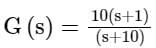for 0 ≤ ω ≤ ∞ will be in the

Detailed Solution for Test: Polar Plot - 1 - Question 1

Given transfer function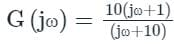(it is Lead compensator)
Magnitude of the transfer function will be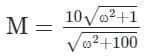Phase of the transfer function will be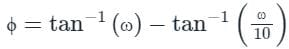At ω = 0
Magnitude M1 = 1 and phase ϕ1 = 0°
At ω = ∞
Magnitude M2 = 10 and phase ϕ2 = 0°
At ω = 2
Magnitude M3 = 2.19 and phase ϕ3 = 52.12°
The polar plot considering the above magnitude and phase values will be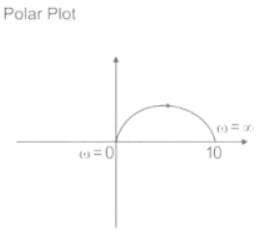Zero is nearer to the imaginary axis than the pole, hence the plot will move in a clockwise direction.

Test: Polar Plot - 1 - Question 2

### System is said to be marginally stable, if

Detailed Solution for Test: Polar Plot - 1 - Question 2

Gain Margin:

• The gain margin is a factor by which the gain of a stable system is allowed to increase before the system reaches the verge of instability.
• It is also defined as the reciprocal of the gain at which the phase angle becomes 1800.
• The frequency at which the phase angle is 1800 is called the phase crossover frequency (ωpc).

Mathematically,
∠G(jω)H(jω) = -180º
If  |G(jω)H(jω)| = a, at ω = ωpc
Then Gain margin,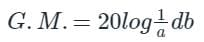Phase Margin:

• It is the amount of additional phase lag (at gain crossover frequency) that is required to bring the system to the verge of instability.
• The frequency at which |G(jω)H(jω|, the magnitude of the open-loop transfer function is unity is called gain crossover frequency (ωpc).

At ωpc, |G(jω)H(jω| = 1
if, ∠G(jω)H(jω) = ϕ at ω = ωgc
Then, P.M. = 180 + ϕ
Analysis: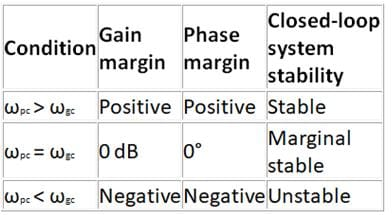For stable systems,
|G(jωpc)H(jωpc| < 1, and
∠G(jωgc)H(jωgc) > -180
so that, gain margin and phase margin both are positive
which is possible when ωgc < ωpc
For marginally stable systems,
ωgc = ωpc
Hence option (b) is the correct answer.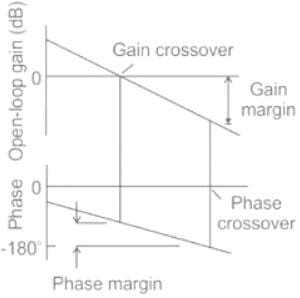Note: For stable systems having two or more gain crossover frequencies, the phase margin is measured at the highest gain crossover frequency.

Test: Polar Plot - 1 - Question 3

### Directions: It consists of two statements, one labelled as the ‘Statement (I)’ and the other as ‘Statement (II)’. Examine these two statements carefully and select the answers to these items using the codes given below: Statement (I): The polar plot has limitation for portraying the frequency response of a system. Statement (II): The calculation of frequency response is tedious and does not indicate the effect of the individual poles and zeros.

Detailed Solution for Test: Polar Plot - 1 - Question 3

Polar plot:

• The Polar plot is a plot, which can be drawn between the magnitude and the phase angle of G(jω)H(jω) by varying ω from zero to ∞.
• The calculation of frequency response is tedious, and it does not indicate the impact of individual components of open-loop transfer function.
• Another disadvantage of this plot is that it cannot tell how many poles are in right side of the complex plane.
• To overcome this disadvantage, magnitude is plotted by varying frequency from that is known as the Nyquist plot.
• It has no limitation in portraying the frequency response of a system.

Therefore, Statement (I) is false but Statement (II) is true.

Test: Polar Plot - 1 - Question 4

Polar plot of transfer function is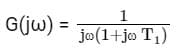Detailed Solution for Test: Polar Plot - 1 - Question 4

Polar plot:
The polar plot is a plot, that is drawn between the magnitude and the phase angle of G(jω) by varying ω from zero 0 to ∞.
Given,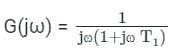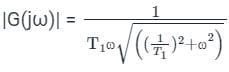∠G(jω) = -90° - tan-1(ωT1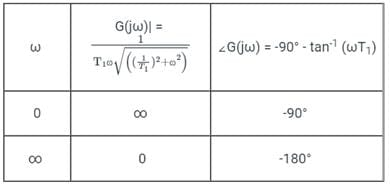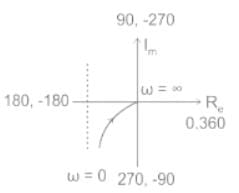Test: Polar Plot - 1 - Question 5

The polar plot of a transfer function with ω as the parameter is known as the

Detailed Solution for Test: Polar Plot - 1 - Question 5

Nyquist plot:
Nyquist plots are an extension of polar plots for finding the stability of the closed-loop control systems. This is done by varying ω from −∞ to ∞, i.e. Nyquist plots are used to draw the complete frequency response of the open-loop transfer function.
Method of drawing Nyquist plot:

• Locate the poles and zeros of open-loop transfer function G(s)H(s) in ‘s’ plane.
• Draw the polar plot by varying ω from zero to infinity.
• Draw the mirror image of the above polar plot for values of ω ranging from −∞ to zero.
• The number of infinite radii half circles will be equal to the number of poles at the origin.
• The infinite radius half-circle will start at the point where the mirror image of the polar plot ends. And this infinite radius half-circle will end at the point where the polar plot starts.
Test: Polar Plot - 1 - Question 6

The transfer function of the following polar plot is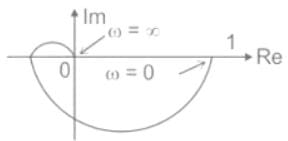Detailed Solution for Test: Polar Plot - 1 - Question 6

From the given polar plot,
At ω = 0, magnitude = 1, angle = 0°
Therefore, there are no poles or zeros at the origin.
At ω = ∞, magnitude = 0, angle = -270°
Therefore, the number of poles = 3
From the above two conditions, the transfer function will be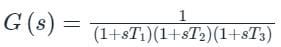Test: Polar Plot - 1 - Question 7

The frequency response of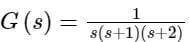plotted in the complex G(jω) plane (for 0 < ω < ∞) is

Detailed Solution for Test: Polar Plot - 1 - Question 7

The given frequency response is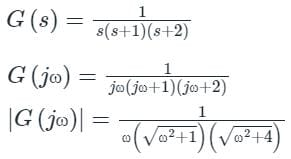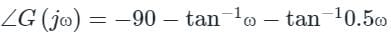In Anti-clockwise the phase order is 0, 90, 180, 270, 360 or 0.
In clockwise the phase angle order is 0, -90, -180, -270, -360 or 0.
Here, 90∘  = -270∘  and 270∘ = -90∘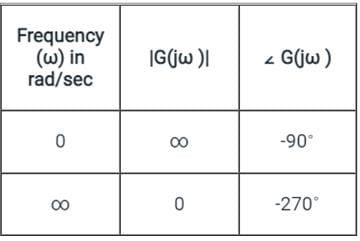At ω = ωc (Cut-off frequency), ∠ G(jω) = -180∘
−90 − tan−1ωc − tan−10.5ωc = −180
tan−1ωc + tan−10.5ωc = 90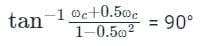1 - 0.5ωc2 = 0
At ω = ωc
|G(jω)| = (1/6)
The polar plot for given transfer function is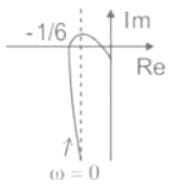Test: Polar Plot - 1 - Question 8

The polar plot of the transfer function G (s) = 10 (s + 1) / (s + 10) will be in the

Detailed Solution for Test: Polar Plot - 1 - Question 8

Polar plot:
The Polar plot is a plot, which can be drawn between the magnitude and the phase angle of the transfer function by varying frequency from 0 to ∞.
Given,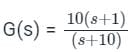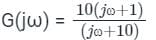the magnitude of the transfer function will be: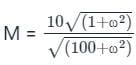The phase of the transfer function will be:
ϕ = tan-1(ω) - tan-1ω(ω/10)
At ω = 0
M1 = 1
ϕ1 = 0°
At ω = ∞
M2 = 10
ϕ2 = 0°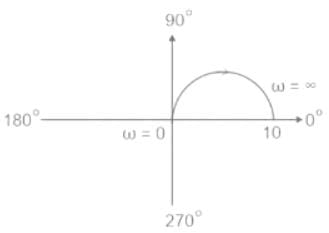Test: Polar Plot - 1 - Question 9

If the GM of the system is 23.9dB then find the value of K.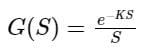Detailed Solution for Test: Polar Plot - 1 - Question 9

The gain margin of the system is 23.9 dB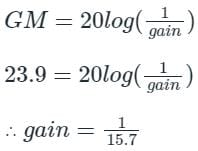∴ phase crossover frequency Wpc = 15.7 rad
∴ at Wpc = 15.7 rad we will get the phase of -180º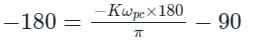-180º = -K(900) -90º
∴ K = 90/900
= 0.1

Test: Polar Plot - 1 - Question 10

Polar plot of transfer function is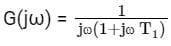Detailed Solution for Test: Polar Plot - 1 - Question 10

Polar plot:
The polar plot is a plot, that is drawn between the magnitude and the phase angle of G(jω) by varying ω from zero 0 to ∞.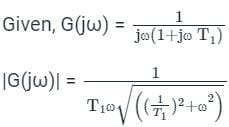∠G(jω) = -90° - tan-1(ωT1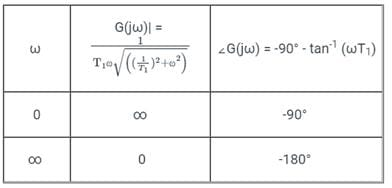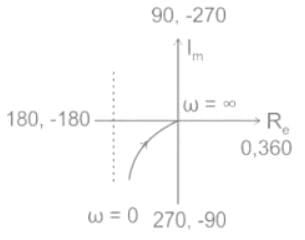Test: Polar Plot - 1 - Question 11

The quadrant at which the polar plot of transfer function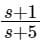lies is ______

Detailed Solution for Test: Polar Plot - 1 - Question 11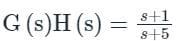(∴ Polar plot lies in 1st quadrant)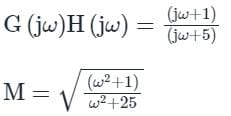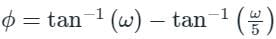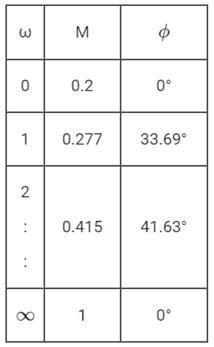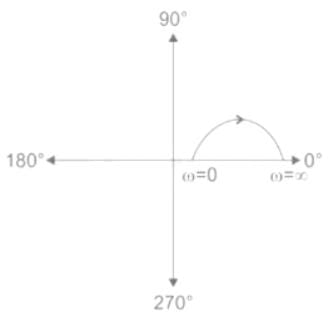Test: Polar Plot - 1 - Question 12

A system with a unity gain margin and zero phase margin is _____

Detailed Solution for Test: Polar Plot - 1 - Question 12
1. Gain margin (GM): The gain margin of the system defines by how much the system gain can be increased so that the system moves on the edge of stability.
2. It is determined from the gain at the phase cross-over frequency.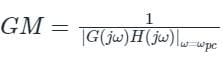3. Phase crossover frequency (ωpc): It is the frequency at which the phase angle of G(s) H(s) is -180°.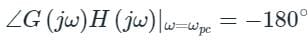4. Phase margin (PM): The phase margin of the system defines by how much the phase of the system can increase to make the system unstable.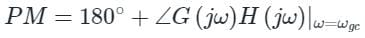5. It is determined from the phase at the gain cross over frequency.
6. Gain crossover frequency (ωgc): It is the frequency at which the magnitude of G(s) H(s) is unity.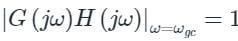7. So it is important to note that these margins of stability are valid for open-loop stable systems only.
8. A large gain margin or a large phase margin indicates a very stable feedback system but usually a very sluggish response.
9. Hence a Gain margin close to unity (or 0 in dB) or a phase margin close to zero corresponds to a highly oscillatory system.

Hence option (3) is the correct answer.
Gain margin and phase margin are frequently used for frequency response specifications by designers. Usually a Gain margin of about 6 dB or a Phase margin of 30 - 35° results in a reasonably good degree of relative stability.
Important Points

• If both GM and PM are positive, the system is stable (ωgc < ωpc)
• If both GM and PM are negative, the system is unstable (ωgc > ωpc)
• If both GM and PM are zero, the system is just stable (ωgc = ωpc)
Test: Polar Plot - 1 - Question 13

Polar plot of sinusoidal transfer function is a plot of:

Detailed Solution for Test: Polar Plot - 1 - Question 13

Polar plot:

• The polar plot of a transfer function G(jω) is the plot of the magnitude of G(jω) versus the phase angle of G(jω) as ω is varied from 0 to positive infinity.
• For all pole systems, type indicates the starting point of the polar plot and order indicates the ending point of the polar plot.

The sinusoidal transfer function G(jω) is a complex function and it is given by
G(jω) = Re [G(jω)] + j Im [G(jω)]
G(jω) = |G(jω)| ∠ G(jω)
= M ∠ϕ Polar form
From the above equation,
G(jω) may be represented as a phasor of magnitude M and phase angle ϕ
The starting points (ω = 0) of a polar plot for different types of minimum phase systems is given below: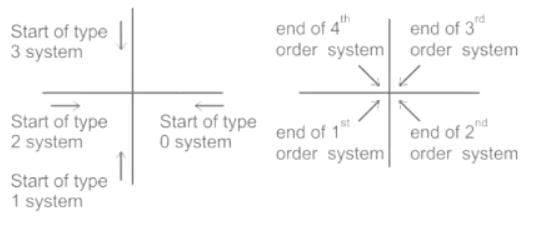• As seen from the above figure, when a zero is added the type decreases, and the end of the polar plot shifts by +90°.
• When a pole is added, the type of the system increases, and hence the end of the polar plot shifts by -90°.

Therefore, the Polar plot of the sinusoidal transfer function is a plot of magnitude and phase angle.

Test: Polar Plot - 1 - Question 14

If the constant 'k' is negative, then what would be its contribution to the phase plot:

Detailed Solution for Test: Polar Plot - 1 - Question 14

Polar plot:

• The polar plot of a transfer function G(jω) is the plot of the magnitude of G(jω) versus the phase angle of G(jω) as ω is varied from 0 to positive infinity.
• For all pole systems, type indicates the starting point of the polar plot and order indicates the ending point of the polar plot.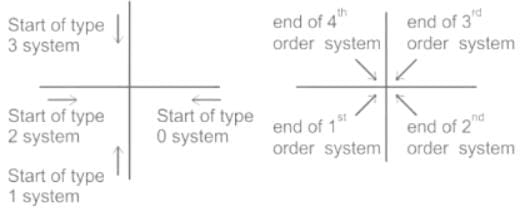• As seen from the above figure, when a zero is added the type decreases, and the end of the polar plot shifts by +90°.
• When a pole is added, the type of the system increases, and hence the end of the polar plot shifts by -90°.

Calculation:
Let the transfer function be: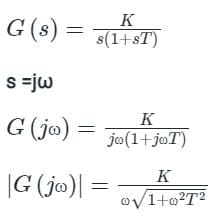The total phase shift will be:
∠G(jω)H(jω) = -90° - tan-1(ωT) - 180°   ----(1)
From equation (1) we can say that constant K has no contribution to the phase plot.
Hence K contributes 180° to the phase plot.
So option (c) is the correct answer.

*Answer can only contain numeric values
Test: Polar Plot - 1 - Question 15

Transfer function of a system is given as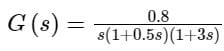Polar plot of the same system is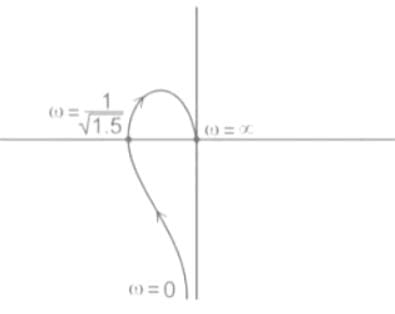Gain margin (in dB) of the system is ______.       (Important - Enter only the numerical value in the answer)

Detailed Solution for Test: Polar Plot - 1 - Question 15

Gain margin (GM) and Phase margin (PM) from the polar plot:
Let us consider the polar plot as shown below,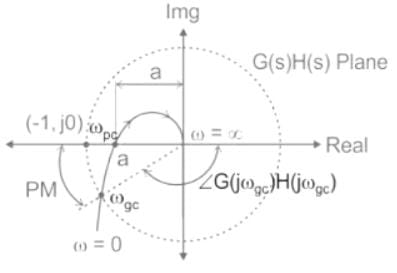Figure: A polar plot of a stable system
Gain margin (GM):
The frequency at which the polar plot crosses the negative real axis is called a phase cross-over frequency (ωpc).
Let 'a' be the point of intersection and it is the magnitude at the phase cross-over frequency.
⇒ a = | G(jωpc) H(jωpc) |
And, we know that
Gain margin (GM) = 1 / |G(jωpc) H(jωpc)| = 1 / a
Gain margin in dB = 20 log (1 / a)
Calculations:
​In the Given polar plot;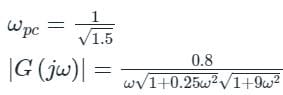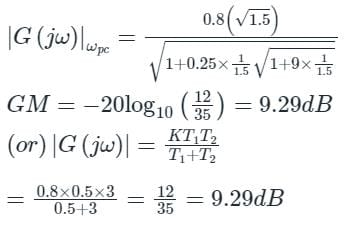Phase margin (PM):
The frequency at which the polar plot crosses the unit circle (formed with the radius of the critical point) is called again cross-over frequency (ωgc).
From the polar plot, we can observe that
Phase margin (PM) = 180 - ∠G(jωgc) H(jωgc​)

## GATE Electrical Engineering (EE) 2023 Mock Test Series

22 docs|274 tests
Information about Test: Polar Plot - 1 Page
In this test you can find the Exam questions for Test: Polar Plot - 1 solved & explained in the simplest way possible. Besides giving Questions and answers for Test: Polar Plot - 1, EduRev gives you an ample number of Online tests for practice

## GATE Electrical Engineering (EE) 2023 Mock Test Series

22 docs|274 tests(Scan QR code)